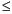Functions and CALL Routines

# LPERM Function

Computes the logarithm of the PERM function, which is the logarithm of the number of permutations of n objects, with the option of including r number of elements.
 Category: Combinatorial

## Syntax

 LPERM(n<,r>)

### Arguments

n

is an integer that represents the total number of elements from which the sample is chosen.

r

is an optional integer value that represents the number of chosen elements. If r is omitted, the function returns the factorial of n.

 Restriction: rn

The LPERM function computes the logarithm of the PERM function.

The following statements produce these results:

SAS Statements Results
x=lperm(5000,500);

put x;

4232.7715946

y=lperm(100,10);

put y;

45.586735935

 Functions:Previous Page | Next Page | Top of Page